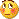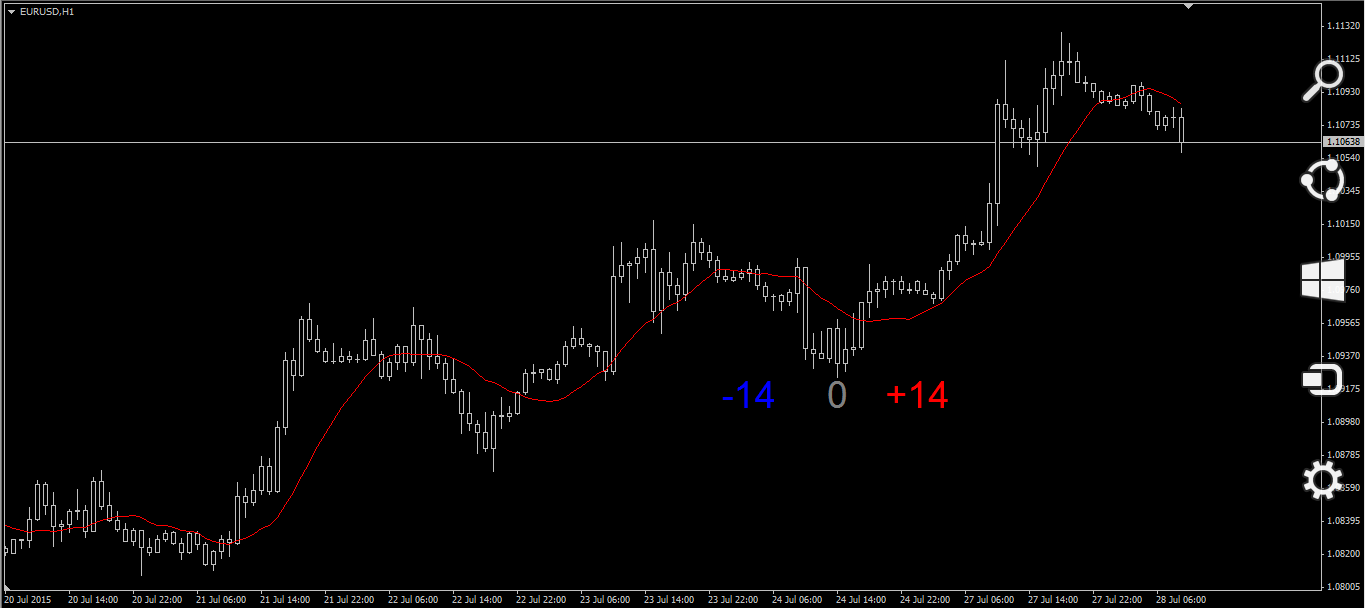# XP Moving Average! - page 53363
hit-the-target

you are generous man !

thank you very much27

To any expert,

We all knew that the moving average is formed by computing the average price of a security over a specific number of periods. My question is, is that any moving average indicator that can calculate a specific day for example period 5, and the first calculate start from the 5th, 10th, 15th, 20th, and 25th day candle all plus together divide by 5 and form a moving average? I'm kind of curious why the indicator only can calculate the continuous days but not the specific days?? Will the moving average become smoother?183056

masshu:
To any expert, We all knew that the moving average is formed by computing the average price of a security over a specific number of periods. My question is, is that any moving average indicator that can calculate a specific day for example period 5, and the first calculate start from the 5th, 10th, 15th, 20th, and 25th day candle all plus together divide by 5 and form a moving average? I'm kind of curious why the indicator only can calculate the continuous days but not the specific days?? Will the moving average become smoother?

masshu

Never saw something like that

One probable reason : if you try to get 5 days at boundaries like that some 5 day periods will be 3 day periods or 4 day periods (weekends) and that would make it unpredictable1202

I think he intend to put the last 5 Fridays together and divide it by 5. For quiet static systems it's ok or at the university, when the result doesn't fit to your hypothesis - throw it away and do so as there are no bad results. Thus Forex is a high dynamic system you can't take out single or more samples. You have to take all in a row. There can happen a lot in 4 days (see EURCHF on 15th January this year).183056

masshu

Never saw something like that

One probable reason : if you try to get 5 days at boundaries like that some 5 day periods will be 3 day periods or 4 day periods (weekends) and that would make it unpredictable

There is a similar thing (Marney indicators Marney Indicators™ | Marney Capital) when just values that exactly match the time of the bar are used for averages, but that was made for volumes and range : I do not believe that it would be of any use for moving averages where the prices can differ significantly in the times of trending markets and you would get values that would lag significantly compared to the current value

That way of averaging can,more or less, be applied to things that are cyclical in nature. Price is not (cyclical in nature)27

And another question I have curious all the time, why does moving average unable insert a negative value? Assuming the price is zero and the red moving average (period +14), we always have the DELAY moving average. If there are negative moving average would it be in ADVANCE? EXCEPT by using shift. I'm still a newbie in trading but i wish someone can explain for me.Files:
untitled.png 32 kb183056

masshu:

And another question I have curious all the time, why does moving average unable insert a negative value? Assuming the price is zero and the red moving average (period +14), we always have the DELAY moving average. If there are negative moving average would it be in ADVANCE? EXCEPT by using shift. I'm still a newbie in trading but i wish someone can explain for me.masshu

It would need to know the future data in that case - and that is impossible27

masshu It would need to know the future data in that case - and that is impossible

What i mean is by using the current data to calculate the negative period of moving average. Example period 5 and the price are 11+12+13+14+15/5=13 of the average but is that possible same period but we add the price with the negative (-11)+(-12)+(-13)+(-14)+(-15)/5=-13??? In mathematics we have positive and negative value/price to draw the positive graph and also negative. But in MT4 would it work?183056

masshu:
mladen What i mean is by using the current data to calculate the negative period of moving average. Example period 5 and the price are 11+12+13+14+15/5=13 of the average but is that possible same period but we add the price with the negative (-11)+(-12)+(-13)+(-14)+(-15)/5=-13??? In mathematics we have positive and negative value/price to draw the positive graph and also negative. But in MT4 would it work?

It is possible - simply multiply the result by -1. But metatrader will not show negative values on the main chart, you have to make it display in a separate window27